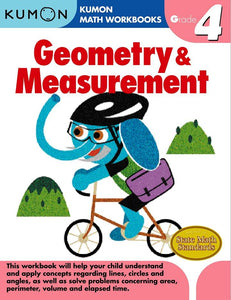# Geometry & Measurement: Grade 4

Regular price
\$8.99
Sale price
\$8.99
Regular price
Out of Stock
Unit price
per

Your child will progress gradually through our activities and master area, volume, circumference, and diameter. Children learn to measure angles with a protractor and calculate decimal and fraction problems with ease.

• Read and write fractions on a number line
• Read and write decimals on a number line
• Understand and apply concepts of lines, angles, and circles
• Draw two-dimensional figures
• Measure and find the area and perimeter of rectangular shapes using appropriate units.
• Measure volume using standard cubic units.
• Measure surface area of cubes and rectangular prisms by adding up the area of the faces
• Calculate elapsed time in hours and half hours
• Calculate elapsed time in days and weeks